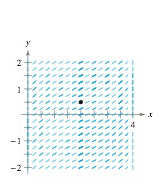Chapter 8.1, Problem 48E

Chapter
Section
Textbook Problem

# Slope Field In Exercises 47 and 48, a differential equation, a point, and a slope field are given, (a) Sketch two approximate solutions of the differential equation on the slope field, one of which passes through the given point. (To print an enlarged copy of the graph, go to McthGraphs.com.) (b) Use integration and the given point to find the particular solution of the differential equation and use a graphing utility to graph the solution. Compare the result with the sketch in part (a) that passes through the given point. d y d x = 1 4 x − x 2 , ( 2 , − 1 2 )(a)

To determine

To calculate: The solution of the differential equation on the slope field.

Explanation

Given:

dydx=14xx2,(2,12) And the slope field,

Calculation:

Consider the differential equation,

dydx=14xx2

Solve,

dydx=14xx2dy=14xx2dx

The above equation if of variable separable form, i

(b)

To determine

To calculate: The particular solution using graphing utility.

### Still sussing out bartleby?

Check out a sample textbook solution.

See a sample solution

#### The Solution to Your Study Problems

Bartleby provides explanations to thousands of textbook problems written by our experts, many with advanced degrees!

Get Started

#### Discuss the continuity of f(x)={ x+21x314x2,3x5.

Calculus: An Applied Approach (MindTap Course List)

#### In Exercises 58, evaluate the expression. 6. |51243|

Applied Calculus for the Managerial, Life, and Social Sciences: A Brief Approach

#### In Problems 7-34, perform the indicated operations and simplify. 34.

Mathematical Applications for the Management, Life, and Social Sciences